# Sine integral

This article is about a particular function from a subset of the real numbers to the real numbers. Information about the function, including its domain, range, and key data relating to graphing, differentiation, and integration, is presented in the article.
View a complete list of particular functions on this wiki
For functions involving angles (trigonometric functions, inverse trigonometric functions, etc.) we follow the convention that all angles are measured in radians. Thus, for instance, the angle of$90\,^\circ$ is measured as$\pi/2$.

## Definition

The function, denoted$\operatorname{Si}$, is defined as the definite integral of the sinc function from 0 to the input point: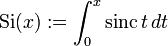$\operatorname{Si}(x) := \int_0^x \operatorname{sinc} \, t \, dt$

Recall that the sinc function is defined in terms of the sine function as follows:$\operatorname{sinc} \, x := \left\lbrace \begin{array}{rl} 1, & x = 0 \\ \frac{\sin x}{x} , & x \ne 0 \\\end{array}\right.$

## Key data

Item Value
default domain all real numbers, i.e., all of$\R$
range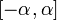$[-\alpha,\alpha]$ where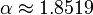$\alpha \approx 1.8519$.
absolute maximum value:$\alpha$, absolute minimum value:$-\alpha$
period none, the function is not periodic
horizontal asymptotes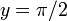$y = \pi/2$ as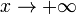$x \to +\infty$ and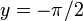$y = -\pi/2$ as$x \to -\infty$.
local maximum values and points of attainment local maximum values are attained at all odd positive integer multiples of$\pi$ and even negative integer multiples of$\pi$. The values differ at each point.
local minimum values and points of attainment local minimum values are attained at all even positive integer multiples of$\pi$ and odd negative integer multiples of$\pi$. The values differ at each point.
points of inflection at$(0,0)$ and at points whose$x$-coordinate is close to an odd multiple of$\pi/2$, except near$\pm \pi/2$.
important symmetries the function is an odd function
derivative] sinc function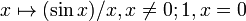$x \mapsto (\sin x)/x, x \ne 0; 1, x = 0$
second derivative$(x \cos x - \sin x)/x^2$ for$x \ne 0$,$0$ for$x = 0$
antiderivative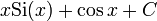$x \operatorname{Si}(x) + \cos x + C$
power series and Taylor series$\sum_{k=0}^\infty \frac{(-1)^kx^{2k+1}}{(2k + 1)(2k + 1)!}$. First few terms:$x - \frac{x^3}{3 \cdot 3!} + \frac{x^5}{5 \cdot 5!} - \dots$

## Differentiation

### First derivative

The first derivative is the sinc function. This follows from the way the sine integral is defined in the first place.

## Integration

### First antiderivative

WHAT WE USE: integration by parts

We have: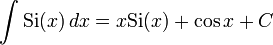$\int \operatorname{Si}(x) \, dx = x \operatorname{Si}(x) + \cos x + C$

We obtain this using integration by parts as follows. We take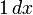$1 \, dx$ as the part to integrate, getting: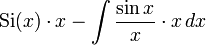$\operatorname{Si}(x) \cdot x - \int \frac{\sin x}{x} \cdot x \, dx$

This simplifies to the desired answer.